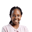Related Tags

scipy
communitycreator
interpolate

# How to use the scipy.interpolate.interp1d() methodJoy Kareko

### Overview

Suppose you have $x$ and $y$ values, and want to use these values to create a linear function where $y=f(x)$. This function can be used to interpolate unknown $y$ values given $x$ values.

In this shot, we’ll examine how to use the scipy.interpolate.interp1d() method to estimate data points of a line by creating a function that already uses two known x and y values. The interp1d means interpolating on a 1 dimension, as in a line, with x and y axes only.

### Syntax

scipy.interpolate.interp1d(x, y, kind = 'linear', axis = - 1, copy = True,
bounds_error = None, fill_value = nan, assume_sorted = False)



### Parameters

• The x and y values are arguments that should be specified when calling this method, but the rest are optional, with the default values as specified.

• The kind parameter specifies the type of curve you want. This parameter can be quadratic, cubic, or any other type but the default is linear.

• The axis specifies the axis along which to interpolate, the default being y.

• The copy parameter makes a copy of x and y first if True or just references x and y if False.

• The bounds_error parameter raises an error every time you try to interpolate an out-of-range value. The error will be ignored if extrapolate is specified in the fill_value parameter.

• The fill_value is NaN by default and NaN values are generated every time you try to interpolate y values out of range unless extrapolate is specified.

• The assume_sorted parameter makes sure that x values are sorted. If True, x values will be values that are increasing.

### Return function

The method returns a function, that can now be used to interpolate y data points.

### Example

import matplotlib.pyplot as plt
import numpy as np
import scipy

from scipy.interpolate import interp1d

x = np.arange(10,20)

print('x:',x)

y = np.exp(-x/10)

print('y:',y)

f_linear = scipy.interpolate.interp1d(x,y)

xnew = np.arange(10,19,0.1)

ynew = f_linear(xnew)

print('new_x:',xnew)
print('new_y:',ynew)

plt.scatter(x, y, color = 'blue')

plt.plot(xnew, ynew, color = 'black')

plt.xlabel("X")

plt.ylabel("Y")

plt.title("1d Interpolation using scipy interp1d method")

plt.show()

plt.savefig('output/graph.png')

### Code explanation

• Lines 1 to 5 import the necessary modules.
• Line 8 generates random points for x using numpy.
• Line 13 generates random points for y using numpy.
• Line 19 creates the linear function for interpolation.
• Line 22 generates new random x points.
• Line 25 interpolates new y points using the linear function generated earlier.
• Lines 10,15,27,28 print out the points generated.
• Lines 32 and 35 plots out scatter and line plots of the points on a graph.
• Lines 38,41 and 45 labels the x and y axes as well as the graph itself.
• Lines 48 and 51 display the graph and saves it respectively.

RELATED TAGS

scipy
communitycreator
interpolate

CONTRIBUTORJoy Kareko
RELATED COURSES

View all Courses

Keep Exploring

Learn in-demand tech skills in half the time